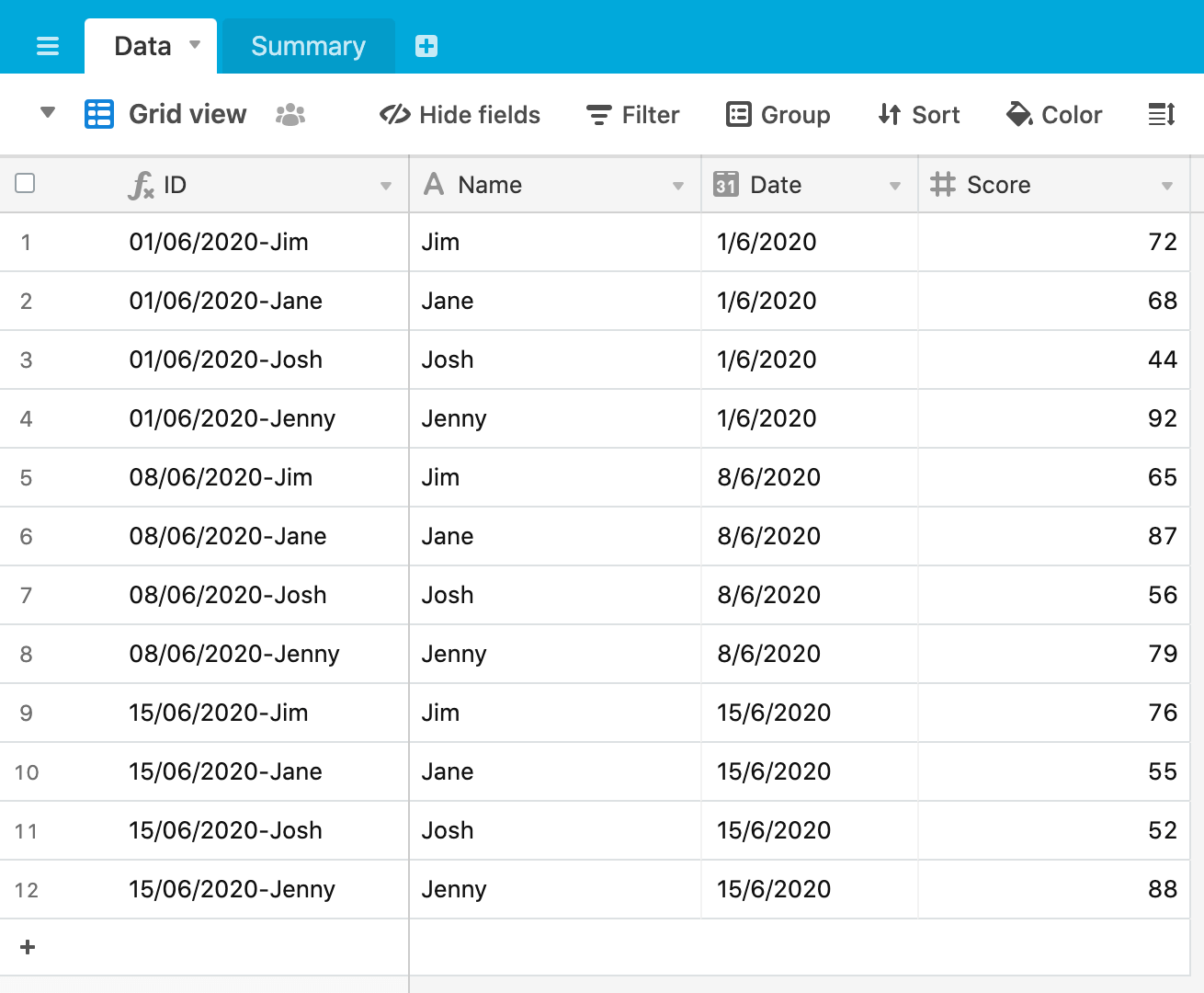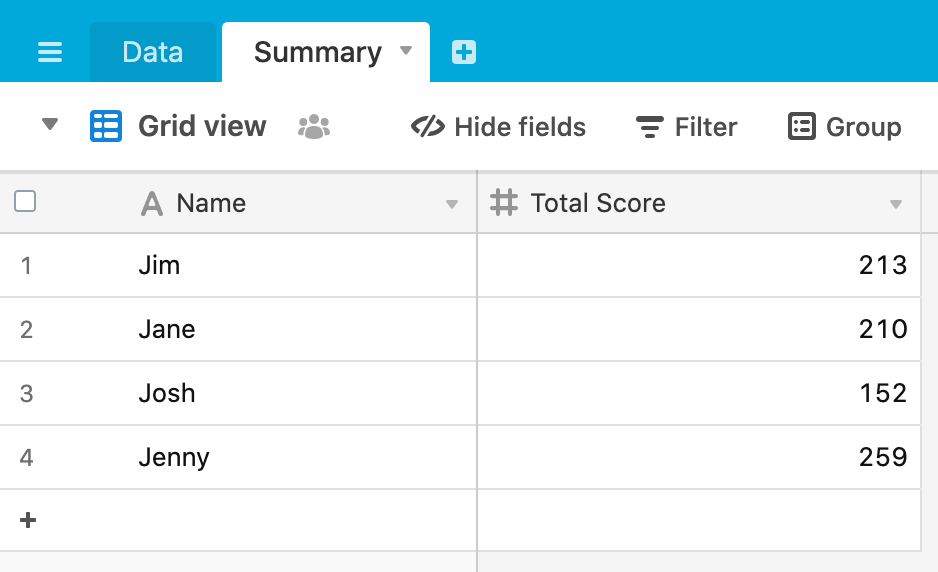### AirscriptLearn to use Airtable scripts

We’ve looked previously at a technique to sum data from your Airtable base. This post looks at another adding up technique - summing data by some attribute of the data. Here’s our scenario:We’ve got a set of scores for a set of people across multiple dates. We want to add these up by person (or in SQL terms, “group by”), to get a total score for each. We want to summarise this data in a second table, ending up with this result.To achieve this we’re going to use the Javascript `reduce` method, so let’s take a quick diversion into `reduce` generally before building our Airtable script. From MDN

“The `reduce()` method executes a reducer function (that you provide) on each element of the array, resulting in single output value.”

(Aside: note that the “single output value” could be an integer, object or an array)

Let’s start with a very simple `reduce` example that sums up the values in an array. Here’s our script (copy this into your Airtable script block):

``````
let array = [ 1, 2, 3, 4 ];
let total = array.reduce(
( accumulator, currentValue ) => accumulator + currentValue,
0
)
console.log(total)

``````

We have an array of integers and we want to total these up. The `reduce` function has two arguments - the `accumulator` and the `currentValue`. The `currentValue` is straightforward - this is the element of the array were currently working with as we iterate through the array. The `accumulator` is the value of the reduce function whose value is remembered across each iteration throughout the array, and ultimately becomes the final, single resulting value.

On the right-hand side of the arrow, we have the function we want to use at each iteration, in this case to add the new `currentValue` to the current value of the `accumulator`. You’ll also notice we have a `0` after the function, which is the initial value of the accumlator. Finally, we `console.log` the total to see the result.

Run the script and you will see, as expected, that the total of 10 is output to the console.

You can play around with some variants on this to get a better feels for what is happening. For example, this script:

``````
let array = [ 1, 2, 3, 4 ];
let total = array.reduce(
(accumulator, currentValue) => accumulator + (currentValue * currentValue),
100
)
console.log(total)

``````

will square each value of the array before adding it to the `accumulator`. Note that we have also set the `accumulator` to have an initial value of `100`, which gives us a result of `130` when the reducer is run. There’s a lot more to reduce functions, so have a look at the examples on MDN and try some out in your script block. Diversion over.

Before we get into our Airtable script, let’s think of the result we need from the `reduce` function to be able to update the Summary table. We need an array of objects of the form:

``````[
...
{
"Name": "Jim",
"Total Score": 100
},
...
]
``````

As we iterate throught the set of records from the Data table, we want to create a new object in our result array if the person doesn’t already exist and, if the person does already exists, we want to add the score from the current iteration to the total score for that person at that point.

Here’s our script:

``````
let dataTbl = base.getTable('Data');
let dataQuery = await dataTbl.selectRecordsAsync();

let result = dataQuery.records.reduce((acc, record) => {
let name = record.getCellValue('Name');
let score = record.getCellValue('Score');
let found = acc.find(item => item['Name'] == name);
if (!found) {
acc.push({'Name': name, 'Total Score': score})
}
else {
found['Total Score'] = found['Total Score'] + score
}
return acc;
}, []);

console.log(result)

``````

Notice in the last line of our `reduce` function that the initial value is an empty array:

` }, []);`

and on each iteration of the function, we’re going to add an object to, or update an existing object in, the array.

The first line of the `reduce` function defines the output (`result`), the array we’re working on (`dataQuery.records`), the accumulator (`acc`) and the variable for each iteration (`record`):

`let result = dataQuery.records.reduce((acc, record) => {`

We then define two variables to hold the values we need from each record:

``````
let name = record.getCellValue('Name');
let score = record.getCellValue('Score');

``````

We then define a variable `found`, where we look through the current state of the `acc` array to see if an object for the current person already exists:

`let found = acc.find(item => item['Name'] == name);`

`array.find()` returns the first element that matches the condition if true or `undefined` if there are zero elements matching the condition.

We then have an `if/else` statement that uses the variable `found`. If `found` is undefined, then we create the object from the current iteration in the `acc` array:

``````
if (!found) {
acc.push({'Name': name, 'Total Score': score})
}

``````

but if `found` returns a record from the `acc` array, we update its total score by adding the `score` of the current iteration to the existing `Total Score`:

``````
else {
found['Total Score'] = found['Total Score'] + score
}

``````

Outputting the result to the console, we get an array of objects, each object with a `Name` and a `Total Score`.

To finish off our script we loop through the `result` array and create a record our Summary table with the data from each result:

``````
let sumTable = base.getTable('Summary');
for (let res of result) {
await sumTable.createRecordAsync(res);
output.text('New summary record created!')
}

``````

As we have already formatted each element of the `result` array to the correct format needed to create a record, we can simply pass in the element to the `createRecordAsync` function.

The full script is:

``````
let dataTbl = base.getTable('Data');
let dataQuery = await dataTbl.selectRecordsAsync();

let result = dataQuery.records.reduce((acc, record) => {
let name = record.getCellValue('Name');
let score = record.getCellValue('Score');
let found = acc.find(item => item['Name'] == name);
if (!found) {
acc.push({'Name': name, 'Total Score': score})
}
else {
found['Total Score'] = found['Total Score'] + score
}
return acc;
}, []);

console.log(result)

let sumTable = base.getTable('Summary');
for (let res of result) {
await sumTable.createRecordAsync(res);
output.text('New summary record created!')
}

``````